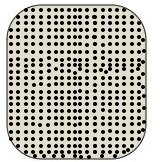# The Equation of State for Real Gases Part Two

## The Reduced VolumeThe number and size of molecules limit their motion inside the container, and the space they cannot reach is referred to as the unavailable volume. For the kinetic theory of gases, where we consider ideal gases, we assume that our molecules are point sized and can take up the entire volume of the container. An example is shown in figure 1. The size, placement, and motion of the molecules are exaggerated, and a large enough number is not shown, but it is easy to picture that extremely small molecules would fill up the space.For the van der Waals model, where we consider real gases, the molecules have an actual size and thus are unable to fill up a container space, even after they collide. An exaggeration of their size and motion is shown in figure 2. The container is the same size as that of figure 1, but the molecules are prevented from inhabiting all of the volume.

In other real gas models, the molecules are further limited in the amount of space they can occupy by a repulsive force that exists between them if they are too close to each other, but we do not consider that here.

From the ideal gas law, we know that

1) PV = nRT

where P is pressure, V is volume, T is temperature, R is the universal gas constant, and n is the number of moles. If we let b equal the amount of the unavailable or excluded volume per mole of the gas, then the actual volume will be the total minus this excluded amount:

2) V (actual) = V – nb

Substituting 2 into equation 1 gives us:

3) P(V – nb) = nRT

Dividing both sides by n we finally have:

4) P(V/n – b) = RT

What does this equation tell us? Suppose we have a set volume V and temperature T. Then the pressure P is greater for our real gas than that of our ideal gas in equation 1. This is because the number of molecules colliding with the walls will increase since the available volume is smaller.

In part two, we will finish our derivation of van der Waals' equation by considering intermolecular forces.

## This post is part of the series: The Equation of State for Real Gases

A basic introduction to van der Waal’s equation of state for real gases.